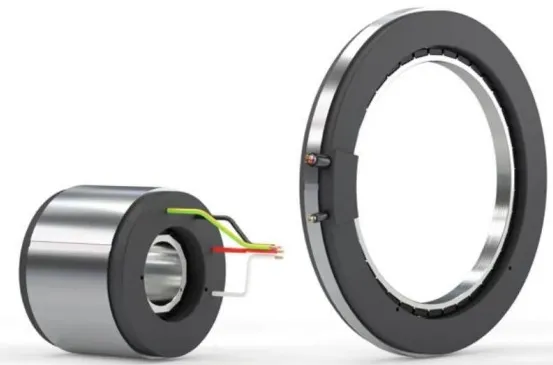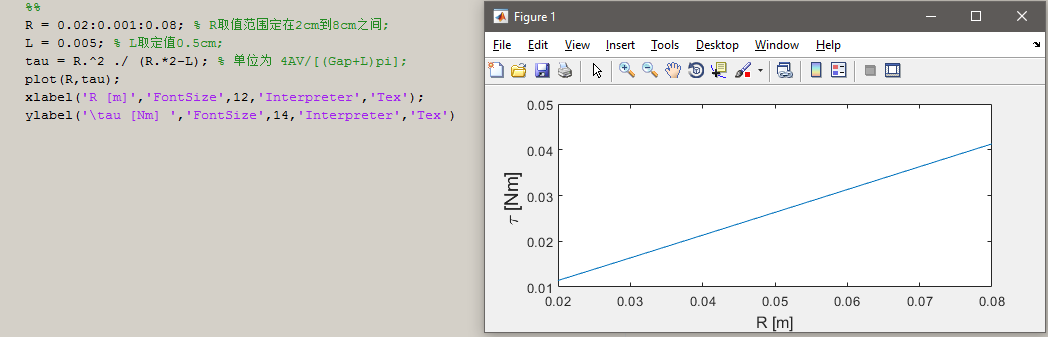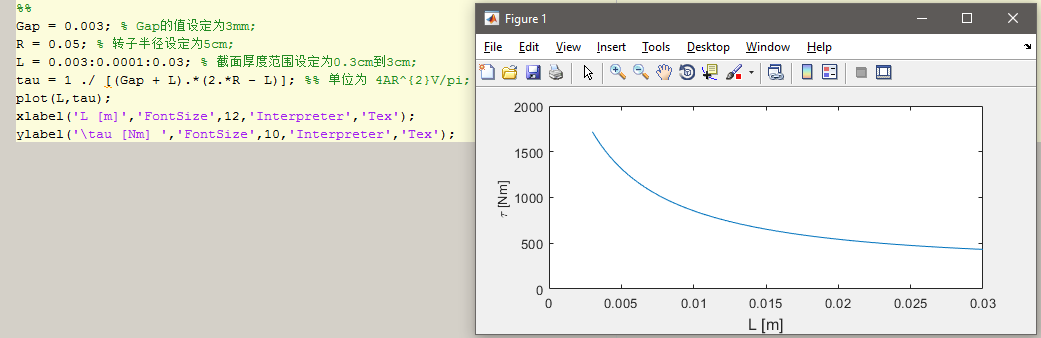# 为什么力矩电机呈现为扁平状？——关于无框力矩电机的一些介绍

(无框)力矩电机，全称应该为永磁无刷直流(无框)力矩电机，其显著的特征是：

• 较大的径长比（diameter-to-length ratio）
• 较多数目的磁极（magnet poles）

• 一般会集成10以内小减速比的减速箱，进一步去符合机器人低速大扭矩的应用特性
• 一般不使用额外的扭矩传感器，通过电流闭环的形式实现力控
• 永磁体可作为内转子（Cheetah初代版本），也可作为外转子（Cheetah Mini版本）输出扭矩均为4Nm的两款无框电机——长筒状 or 扁平状 ？

$$\tau = \frac{R^{2}4\mu{0}k{s}LM_{0}\omega}{Gap+L} ,(1)$$

$$\tau = 4A \cdot \frac{R^{2}L\omega}{Gap+L} ,(2)$$

• 因为我们仅考虑$R$ 与 $\omega$ 取值的关系，作为简化，我们将永磁体截面厚度$L$ 和定转子间隙$Gap$ 暂时设为定量；
• 同时假设永磁体的体积$V$ 一定，永磁体体积有$V = \pi\omega L (2R-L)$ 的关系。假设$V$ 为常量——是在我们设计腿足机器人的电机时非常重要的一点，因为我们永远考虑的都是【扭矩/质量】的输出密度的概念，即我们在比较任何参数给电机输出扭矩带来改变的同时，一定要考虑相应的质量（体积），而最好的做法就是保持质量一定；

$$\tau = 4A\cdot\frac{V}{(Gap+L)\pi}\cdot\frac{R^{2}}{2R-L},(3)$$$$\tau = 4A\cdot\frac{R^{2}V}{\pi}\cdot\frac{1}{(Gap+L)(2R-L)},(4)$$